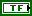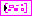# TFA Dual Window VI

LabVIEW 2014 Advanced Signal Processing Toolkit Help

Edition Date: June 2014

Part Number: 372656C-01

»View Product InfoDownload Help (Windows Only)

Owning Palette: Time Frequency Transform VIs

Computes the dual windows for the Gabor transform and the Gabor expansion.equal length? specifies if the lengths of the window function and the signal are equal. When the window length is equal to the signal length, the chance of obtaining the optimal dual function is higher. The default is FALSE. The optimal dual function has a similar shape as the synthesis window. The time-frequency representation might have better concentration with the optimal dual function. However, this VI needs more time and memory to compute the time-frequency representation when the window length and the signal length are large.dM (time steps) specifies the time shift, in samples, between elementary functions. The time sampling interval of the signal in the time-frequency domain is dM (time steps)/fs, where fs is the sampling rate of the signal. National Instruments recommends that you set dM (time steps) to a power of 2.N (frequency bins) specifies the number of frequencies of the Gabor elementary functions. The frequency sampling interval of the signal in the time-frequency plane is fs/N (frequency bins), where fs is the sampling rate of the signal. N (frequency bins) must be a power of 2. For stable reconstruction, N (frequency bins) must be greater than or equal to dM (time steps). The ratio between N (frequency bins) and dM (time steps) is called the oversampling rate.Gaussian synthesis window specifies the length and variance of the Gaussian window of the Gabor elementary functions. Use the user defined synthesis window input to specify other types of envelope widows for the elementary functions.length specifies the length, in samples, of the Gaussian synthesis window. length must be divided evenly by dM (time steps) and N (frequency bins).variance specifies the variance, in square samples, of the Gaussian synthesis window. The default is –1. When the value is -1, this VI calculates the optimal variance, which is equal to N (frequency bins)×dM (time steps)/(2×pi). If Gaussian synthesis window has optimal variance, the linear time-frequency representation of the signal will have the best concentration.error in describes error conditions that occur before this node runs. This input provides standard error in functionality.user defined synthesis window specifies the coefficients of the envelope window to use for the Gabor elementary functions. If you specify a value for user defined synthesis window, this VI ignores the settings in the Gaussian synthesis window input. The size of user defined synthesis window must be divided evenly by dM (time steps) and N (frequency bins).analysis info returns the analysis window that the TFA Discrete Gabor Transform VI uses.analysis window returns the window this VI uses to compute the Gabor coefficients. analysis window is the dual window of the synthesis window, which is the envelope of the Gabor elementary functions. The size of analysis window must be divided evenly by dM (time steps) and N (frequency bins).dM (time steps) returns the time shift, in samples, between elementary functions. The time sampling interval of the signal in the time-frequency domain is dM (time steps)/fs, where fs is the sampling rate of the signal. National Instruments recommends that dM (time steps) be a power of 2.N (frequency bins) returns the number of frequencies of the Gabor elementary functions. The frequency sampling interval of the signal in the time-frequency plane is fs/N (frequency bins), where fs is the sampling rate of the signal. N (frequency bins) must be a power of 2. For stable reconstruction, N (frequency bins) must be greater than or equal to dM (time steps). The ratio between N (frequency bins) and dM (time steps) is called the oversampling rate.synthesis info returns the Gabor elementary functions that the TFA Discrete Gabor Expansion VI uses.synthesis window returns the window of the Gabor elementary functions. The size of synthesis window must be divided evenly by dM (time steps) and N (frequency bins).dM (time steps) returns the time shift, in samples, between elementary functions. The time sampling interval of the signal in the time-frequency domain is dM (time steps)/fs, where fs is the sampling rate of the signal. National Instruments recommends that dM (time steps) be a power of 2.N (frequency bins) returns the number of frequencies of the Gabor elementary functions. The frequency sampling interval of the signal in the time-frequency plane is fs/N (frequency bins), where fs is the sampling rate of the signal. N (frequency bins) must be a power of 2. For stable reconstruction, N (frequency bins) must be greater than or equal to dM (time steps). The ratio between N (frequency bins) and dM (time steps) is called the oversampling rate.error out contains error information. This output provides standard error out functionality.

## TFA Dual Window Details

The synthesis window is the envelope window of the Gabor elementary functions. The dual window of the synthesis window is called the analysis window, which this VI uses in the Gabor transform to compute the complex weight of the Gabor elementary functions. You can use the resulting analysis info and synthesis info for the Gabor transform and the Gabor expansion, respectively.

To characterize the local properties of a signal in the joint time-frequency domain accurately, the analysis window and the synthesis window must match as closely as possible. As shown in the book Introduction to Time-Frequency and Wavelet Transforms, as the oversampling ratio N/dM increases, the dual functions move closer together.

## Example

Refer to the Voice Enhancement VI in the labview\examples\Time Frequency Analysis\TFAApplications directory for an example of using the TFA Dual Window VI.